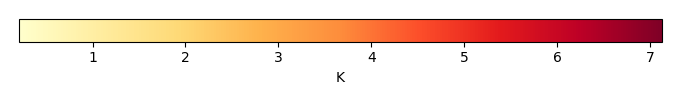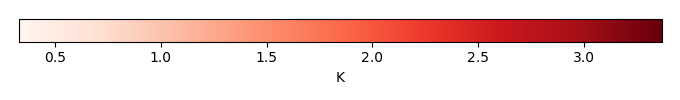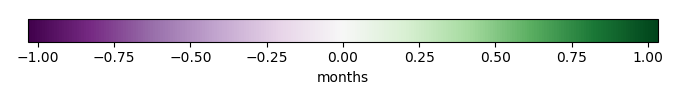# Mean State

Period Mean (original grids) [K]
Model Period Mean (intersection) [K]
Model Period Mean (complement) [K]
Benchmark Period Mean (intersection) [K]
Benchmark Period Mean (complement) [K]
Bias [K]
RMSE [K]
Phase Shift [months]
Bias Score 
RMSE Score 
Seasonal Cycle Score 
Spatial Distribution Score 
Interannual Variability Score 
Overall Score 
Benchmark [-] 299.
CLM5PHSOFF [-] 299. 299. 300. 298. 300. 0.225 0.724 0.428 0.647 0.753 0.963 1.00 0.954 0.845
CLM5PHSON [-] 299. 299. 300. 298. 300. 0.285 0.690 0.366 0.667 0.779 0.967 1.00 0.955 0.858
Period Mean (original grids) [K]
Model Period Mean (intersection) [K]
Model Period Mean (complement) [K]
Benchmark Period Mean (intersection) [K]
Benchmark Period Mean (complement) [K]
Bias [K]
RMSE [K]
Phase Shift [months]
Bias Score 
RMSE Score 
Seasonal Cycle Score 
Spatial Distribution Score 
Interannual Variability Score 
Overall Score 
Benchmark [-] 300.
CLM5PHSOFF [-] 301. 301. 300. 300. 300. 0.460 0.798 0.168 0.747 0.862 0.987 0.989 0.965 0.902
CLM5PHSON [-] 301. 301. 301. 300. 300. 0.473 0.802 0.168 0.746 0.862 0.987 0.990 0.964 0.902
Period Mean (original grids) [K]
Model Period Mean (intersection) [K]
Model Period Mean (complement) [K]
Benchmark Period Mean (intersection) [K]
Benchmark Period Mean (complement) [K]
Bias [K]
RMSE [K]
Phase Shift [months]
Bias Score 
RMSE Score 
Seasonal Cycle Score 
Spatial Distribution Score 
Interannual Variability Score 
Overall Score 
Benchmark [-] 295.
CLM5PHSOFF [-] 296. 296. 297. 295. 297. 0.525 0.859 0.287 0.703 0.871 0.967 0.999 0.962 0.895
CLM5PHSON [-] 296. 296. 296. 295. 297. 0.505 0.854 0.273 0.707 0.867 0.968 0.999 0.960 0.895
Period Mean (original grids) [K]
Model Period Mean (intersection) [K]
Model Period Mean (complement) [K]
Benchmark Period Mean (intersection) [K]
Benchmark Period Mean (complement) [K]
Bias [K]
RMSE [K]
Phase Shift [months]
Bias Score 
RMSE Score 
Seasonal Cycle Score 
Spatial Distribution Score 
Interannual Variability Score 
Overall Score 
Benchmark [-] 262.
CLM5PHSOFF [-] 262. 262. 269. 262. 266. 0.253 1.14 0.0145 0.941 0.956 0.999 1.00 0.938 0.965
CLM5PHSON [-] 262. 261. 267. 262. 266. 0.258 1.13 0.0161 0.941 0.957 0.999 1.00 0.939 0.965
Period Mean (original grids) [K]
Model Period Mean (intersection) [K]
Model Period Mean (complement) [K]
Benchmark Period Mean (intersection) [K]
Benchmark Period Mean (complement) [K]
Bias [K]
RMSE [K]
Phase Shift [months]
Bias Score 
RMSE Score 
Seasonal Cycle Score 
Spatial Distribution Score 
Interannual Variability Score 
Overall Score 
Benchmark [-] 299.
CLM5PHSOFF [-] 299. 299. 300. 299. 299. 0.160 0.746 0.476 0.430 0.710 0.930 0.962 0.913 0.776
CLM5PHSON [-] 299. 299. 300. 299. 299. 0.226 0.750 0.486 0.425 0.725 0.930 0.963 0.913 0.780
Period Mean (original grids) [K]
Model Period Mean (intersection) [K]
Model Period Mean (complement) [K]
Benchmark Period Mean (intersection) [K]
Benchmark Period Mean (complement) [K]
Bias [K]
RMSE [K]
Phase Shift [months]
Bias Score 
RMSE Score 
Seasonal Cycle Score 
Spatial Distribution Score 
Interannual Variability Score 
Overall Score 
Benchmark [-] 271.
CLM5PHSOFF [-] 271. 271. 275. 271. 274. -0.0455 0.709 0.00842 0.966 0.965 0.999 1.00 0.980 0.979
CLM5PHSON [-] 271. 271. 275. 271. 274. -0.0249 0.647 0.00842 0.966 0.971 0.999 1.00 0.979 0.981
Period Mean (original grids) [K]
Model Period Mean (intersection) [K]
Model Period Mean (complement) [K]
Benchmark Period Mean (intersection) [K]
Benchmark Period Mean (complement) [K]
Bias [K]
RMSE [K]
Phase Shift [months]
Bias Score 
RMSE Score 
Seasonal Cycle Score 
Spatial Distribution Score 
Interannual Variability Score 
Overall Score 
Benchmark [-] 280.
CLM5PHSOFF [-] 281. 281. 289. 280. 285. 0.283 1.10 0.0208 0.900 0.956 0.999 1.00 0.966 0.963
CLM5PHSON [-] 281. 280. 288. 280. 285. 0.249 1.09 0.0222 0.901 0.956 0.998 1.00 0.965 0.963
Period Mean (original grids) [K]
Model Period Mean (intersection) [K]
Model Period Mean (complement) [K]
Benchmark Period Mean (intersection) [K]
Benchmark Period Mean (complement) [K]
Bias [K]
RMSE [K]
Phase Shift [months]
Bias Score 
RMSE Score 
Seasonal Cycle Score 
Spatial Distribution Score 
Interannual Variability Score 
Overall Score 
Benchmark [-] 297.
CLM5PHSOFF [-] 297. 297. 300. 297. 300. 0.162 1.07 0.164 0.764 0.879 0.984 1.00 0.950 0.909
CLM5PHSON [-] 297. 297. 300. 297. 300. 0.110 1.08 0.181 0.766 0.877 0.983 1.00 0.946 0.908
Period Mean (original grids) [K]
Model Period Mean (intersection) [K]
Model Period Mean (complement) [K]
Benchmark Period Mean (intersection) [K]
Benchmark Period Mean (complement) [K]
Bias [K]
RMSE [K]
Phase Shift [months]
Bias Score 
RMSE Score 
Seasonal Cycle Score 
Spatial Distribution Score 
Interannual Variability Score 
Overall Score 
Benchmark [-] 264.
CLM5PHSOFF [-] 264. 264. 268. 264. 264. 0.0749 0.718 0.0130 0.967 0.966 0.999 1.00 0.962 0.977
CLM5PHSON [-] 264. 264. 267. 264. 264. 0.0517 0.717 0.0135 0.967 0.966 0.999 1.00 0.963 0.977
Period Mean (original grids) [K]
Model Period Mean (intersection) [K]
Model Period Mean (complement) [K]
Benchmark Period Mean (intersection) [K]
Benchmark Period Mean (complement) [K]
Bias [K]
RMSE [K]
Phase Shift [months]
Bias Score 
RMSE Score 
Seasonal Cycle Score 
Spatial Distribution Score 
Interannual Variability Score 
Overall Score 
Benchmark [-] 295.
CLM5PHSOFF [-] 295. 295. 293. 295. 294. 0.473 0.695 0.0952 0.877 0.927 0.993 1.00 0.969 0.949
CLM5PHSON [-] 295. 295. 294. 295. 294. 0.479 0.699 0.0807 0.876 0.929 0.994 1.00 0.969 0.950
Period Mean (original grids) [K]
Model Period Mean (intersection) [K]
Model Period Mean (complement) [K]
Benchmark Period Mean (intersection) [K]
Benchmark Period Mean (complement) [K]
Bias [K]
RMSE [K]
Phase Shift [months]
Bias Score 
RMSE Score 
Seasonal Cycle Score 
Spatial Distribution Score 
Interannual Variability Score 
Overall Score 
Benchmark [-] 282.
CLM5PHSOFF [-] 282. 282. 285. 282. 286. 0.175 0.883 0.126 0.897 0.935 0.992 1.00 0.970 0.955
CLM5PHSON [-] 282. 282. 284. 282. 286. 0.117 0.866 0.145 0.899 0.936 0.990 1.00 0.970 0.955
Period Mean (original grids) [K]
Model Period Mean (intersection) [K]
Model Period Mean (complement) [K]
Benchmark Period Mean (intersection) [K]
Benchmark Period Mean (complement) [K]
Bias [K]
RMSE [K]
Phase Shift [months]
Bias Score 
RMSE Score 
Seasonal Cycle Score 
Spatial Distribution Score 
Interannual Variability Score 
Overall Score 
Benchmark [-] 287.
CLM5PHSOFF [-] 283. 287. 262. 287. 292. 0.236 0.910 0.143 0.828 0.904 0.985 1.00 0.960 0.930
CLM5PHSON [-] 283. 287. 249. 287. 292. 0.227 0.898 0.137 0.829 0.907 0.986 1.00 0.959 0.931
Period Mean (original grids) [K]
Model Period Mean (intersection) [K]
Model Period Mean (complement) [K]
Benchmark Period Mean (intersection) [K]
Benchmark Period Mean (complement) [K]
Bias [K]
RMSE [K]
Phase Shift [months]
Bias Score 
RMSE Score 
Seasonal Cycle Score 
Spatial Distribution Score 
Interannual Variability Score 
Overall Score 
Benchmark [-] 296.
CLM5PHSOFF [-] 296. 296. 296. 296. 297. 0.214 0.830 0.131 0.898 0.939 0.990 0.999 0.964 0.955
CLM5PHSON [-] 296. 296. 296. 296. 297. 0.191 0.824 0.134 0.898 0.939 0.990 0.999 0.963 0.955
Period Mean (original grids) [K]
Model Period Mean (intersection) [K]
Model Period Mean (complement) [K]
Benchmark Period Mean (intersection) [K]
Benchmark Period Mean (complement) [K]
Bias [K]
RMSE [K]
Phase Shift [months]
Bias Score 
RMSE Score 
Seasonal Cycle Score 
Spatial Distribution Score 
Interannual Variability Score 
Overall Score 
Benchmark [-] 265.
CLM5PHSOFF [-] 265. 265. 267. 265. 266. 0.262 0.982 0.0180 0.944 0.951 0.999 1.00 0.958 0.967
CLM5PHSON [-] 265. 265. 266. 265. 266. 0.255 0.978 0.0188 0.944 0.951 0.999 1.00 0.959 0.967
Period Mean (original grids) [K]
Model Period Mean (intersection) [K]
Model Period Mean (complement) [K]
Benchmark Period Mean (intersection) [K]
Benchmark Period Mean (complement) [K]
Bias [K]
RMSE [K]
Phase Shift [months]
Bias Score 
RMSE Score 
Seasonal Cycle Score 
Spatial Distribution Score 
Interannual Variability Score 
Overall Score 
Benchmark [-] 296.
CLM5PHSOFF [-] 296. 296. 299. 296. 298. 0.406 1.19 0.246 0.666 0.857 0.979 0.997 0.949 0.884
CLM5PHSON [-] 296. 295. 298. 296. 298. 0.401 1.19 0.241 0.667 0.862 0.979 0.997 0.945 0.885
Period Mean (original grids) [K]
Model Period Mean (intersection) [K]
Model Period Mean (complement) [K]
Benchmark Period Mean (intersection) [K]
Benchmark Period Mean (complement) [K]
Bias [K]
RMSE [K]
Phase Shift [months]
Bias Score 
RMSE Score 
Seasonal Cycle Score 
Spatial Distribution Score 
Interannual Variability Score 
Overall Score 
Benchmark [-] 286.
CLM5PHSOFF [-] 287. 287. 293. 286. 292. 0.325 0.636 0.0307 0.940 0.958 0.998 1.00 0.974 0.971
CLM5PHSON [-] 286. 286. 294. 286. 292. 0.281 0.608 0.0307 0.942 0.960 0.998 1.00 0.973 0.972
Period Mean (original grids) [K]
Model Period Mean (intersection) [K]
Model Period Mean (complement) [K]
Benchmark Period Mean (intersection) [K]
Benchmark Period Mean (complement) [K]
Bias [K]
RMSE [K]
Phase Shift [months]
Bias Score 
RMSE Score 
Seasonal Cycle Score 
Spatial Distribution Score 
Interannual Variability Score 
Overall Score 
Benchmark [-] 275.
CLM5PHSOFF [-] 275. 275. 279. 274. 278. 0.104 0.719 0.0418 0.943 0.955 0.997 0.999 0.972 0.970
CLM5PHSON [-] 274. 274. 278. 274. 278. 0.109 0.688 0.0418 0.943 0.959 0.997 0.999 0.972 0.971
Period Mean (original grids) [K]
Model Period Mean (intersection) [K]
Model Period Mean (complement) [K]
Benchmark Period Mean (intersection) [K]
Benchmark Period Mean (complement) [K]
Bias [K]
RMSE [K]
Phase Shift [months]
Bias Score 
RMSE Score 
Seasonal Cycle Score 
Spatial Distribution Score 
Interannual Variability Score 
Overall Score 
Benchmark [-] 284.
CLM5PHSOFF [-] 284. 284. 287. 284. 286. 0.380 1.13 0.0293 0.879 0.933 0.998 1.00 0.964 0.951
CLM5PHSON [-] 284. 284. 287. 284. 286. 0.371 1.11 0.0268 0.879 0.936 0.998 1.00 0.963 0.952
Period Mean (original grids) [K]
Model Period Mean (intersection) [K]
Model Period Mean (complement) [K]
Benchmark Period Mean (intersection) [K]
Benchmark Period Mean (complement) [K]
Bias [K]
RMSE [K]
Phase Shift [months]
Bias Score 
RMSE Score 
Seasonal Cycle Score 
Spatial Distribution Score 
Interannual Variability Score 
Overall Score 
Benchmark [-] 299.
CLM5PHSOFF [-] 299. 299. 300. 299. 299. 0.120 0.712 0.439 0.656 0.756 0.943 0.998 0.924 0.839
CLM5PHSON [-] 299. 299. 300. 299. 299. 0.168 0.688 0.391 0.654 0.785 0.948 0.999 0.925 0.849
Period Mean (original grids) [K]
Model Period Mean (intersection) [K]
Model Period Mean (complement) [K]
Benchmark Period Mean (intersection) [K]
Benchmark Period Mean (complement) [K]
Bias [K]
RMSE [K]
Phase Shift [months]
Bias Score 
RMSE Score 
Seasonal Cycle Score 
Spatial Distribution Score 
Interannual Variability Score 
Overall Score 
Benchmark [-] 296.
CLM5PHSOFF [-] 296. 296. 296. 296. 293. 0.410 0.678 0.236 0.760 0.877 0.971 1.00 0.964 0.908
CLM5PHSON [-] 296. 296. 296. 296. 293. 0.383 0.666 0.220 0.763 0.880 0.973 1.00 0.963 0.910
Period Mean (original grids) [K]
Model Period Mean (intersection) [K]
Model Period Mean (complement) [K]
Benchmark Period Mean (intersection) [K]
Benchmark Period Mean (complement) [K]
Bias [K]
RMSE [K]
Phase Shift [months]
Bias Score 
RMSE Score 
Seasonal Cycle Score 
Spatial Distribution Score 
Interannual Variability Score 
Overall Score 
Benchmark [-] 284.
CLM5PHSOFF [-] 285. 284. 288. 284. 285. 0.513 1.56 0.189 0.659 0.861 0.977 1.00 0.951 0.885
CLM5PHSON [-] 285. 284. 288. 284. 285. 0.483 1.54 0.183 0.661 0.862 0.977 1.00 0.952 0.886

# Temporally integrated period mean

BENCHMARK MEANMODEL MEANBIASBIAS SCORERMSERMSE SCOREBENCHMARK INTERANNUAL VARIABILITYMODEL INTERANNUAL VARIABILITYINTERANNUAL VARIABILITY SCOREBENCHMARK MAX MONTHMODEL MAX MONTHDIFFERENCE IN MAX MONTHSEASONAL CYCLE SCORESPATIAL TAYLOR DIAGRAMMODEL COLORS# Spatially integrated regional mean

MODEL COLORSREGIONAL MEANANNUAL CYCLEMONTHLY ANOMALYANNUAL CYCLE# All Models

BenchmarkCLM5PHSOFFCLM5PHSON# Data Information

creation_date: Fri Nov 1 11:52:30 PDT 2013

source_file: This product is generated from monthly CRU TS3.21 Mean Temperature observations

title: derived CRU high resolution (0.5x0.5) Mean Temperature

Approach: I simply read the monthly surface air temperature from original file, and separated the time series by a month and saved them by each month and each year.

Temporal resolution: monthly

General information: This product was derived from CRU v3.21 product.

Spatial resolution: 0.5x0.5 degree